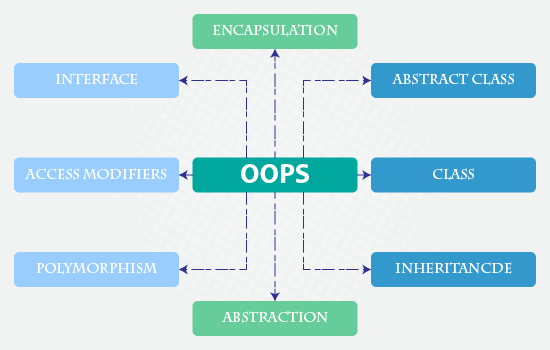Object-Oriented PHP with Example for Beginners - Mostlikers

## 26 April, 2017

### Object-Oriented PHP with Example for Beginners

Today we are going to discuss on basics of object-oriented programming in PHP. For many PHP programmers struggling to learn object-oriented logic. When I was started to learn the oops concept. I don't know why need to use the oops concept in PHP?. Later I have read so many tutorials, I got some good example and easy way to learn oops concept. In this tutorial, I have shared the oops concept with some sample programs.Before going learn we need to know about why should we use oops in PHP?
• Object-oriented programming (OOP) is a programming paradigm based on the concept of "objects", which may contain data, in the form of fields, often known as attributes; and code, in the form of procedures, often known as methods.
• OOP is a style of coding that allows developers to group similar tasks into classes.

### Step by step Process learn oops

Before we go into detail, let's define some important terms related to OOPS.
• Class -  A class is a template definition of the methods and variables in a particular kind of object.
• Object -  Object refers to a particular instance of a class where the object can be a combination of variables, functions, and data structures.

### How to Create Class and Objects

The following syntax contains the create a new class in PHP
```<?php
class MyClass
{
//Class property and methods
}
?>
```
• You can define any name in the class. The syntax define the class name as Myclass

### Create Object in PHP

For access, the class function and method create an object by using a new keyword with the class name. Outside of the class.
```<?php

class MyClass
{
//Class property and methods
}

new MyClass();
?>
```

### Example

Here some sample program to easy way learns class and objects in PHP.

```<?php

class student
{
//Class Functions
public function name(\$name)
{
return \$name; // User input name
}

public function number()
{
\$rand = rand(); // some random number
return \$rand; // Return the result
}
}

\$program = new student(); // Class methods access

\$student_name = \$program->name('Karthick'); // user input

\$student_number = \$program->number();

echo 'Student name is '.\$student_name.'</br>';

echo 'Student number is '.\$student_number;
?>
```

#### Output will produce the following result

```Student name is Karthick
Student number is 28984
```

• The student is a new class name. Inside the class name, we define two public function name, number.
• function name(\$name) -  \$name is a dynamic argument. The data object \$program->name('karthick'); you can pass whatever text you want.
• \$program->number()  - Calling member function to out side the class.

### Calling Member Function and Member Variable

• Member Variable - This variable defines inside the class. It won't visible outside of the class.

#### Member Function - These are the function defined inside a class. It can access objective data. Example

Simple example program
```<?php
class students {
// Member variables //
var \$name;
var \$rollnumber;

// Member functions //
function setname(\$name){
\$this->name = \$name;
}

function setrollnumber(\$number){
\$this->rollnumber = \$number;
}

function getname(){
echo \$this->name ."<br/>";
}

function getrollnumber(){
echo \$this->rollnumber ." <br/>";
}
}

\$school = new students();
\$school->setname('Karthick'); // access member variables
\$school->setrollnumber('77'); // access member variables

// Access the member function

echo \$school->getname();
echo \$school->getrollnumber();

?>
```

OUTPUT
```Karthick
77
```

This section we just shared basic information about oops concept. The upcoming tutorial we will guide you OOPS function with Some live example script. Hope you like this tutorial, Drop your comments.